﻿ Python: Slice a tuple - w3resource# Python Exercise: Slice a tuple

## Python tuple: Exercise-13 with Solution

Write a Python program to slice a tuple.

Sample Solution:-

Python Code:

``````#create a tuple
tuplex = (2, 4, 3, 5, 4, 6, 7, 8, 6, 1)
#used tuple[start:stop] the start index is inclusive and the stop index
_slice = tuplex[3:5]
#is exclusive
print(_slice)
#if the start index isn't defined, is taken from the beg inning of the tuple
_slice = tuplex[:6]
print(_slice)
#if the end index isn't defined, is taken until the end of the tuple
_slice = tuplex[5:]
print(_slice)
#if neither is defined, returns the full tuple
_slice = tuplex[:]
print(_slice)
#The indexes can be defined with negative values
_slice = tuplex[-8:-4]
print(_slice)
#create another tuple
tuplex = tuple("HELLO WORLD")
print(tuplex)
#step specify an increment between the elements to cut of the tuple
#tuple[start:stop:step]
_slice = tuplex[2:9:2]
print(_slice)
#returns a tuple with a jump every 3 items
_slice = tuplex[::4]
print(_slice)
#when step is negative the jump is made back
_slice = tuplex[9:2:-4]
print(_slice)
```
```

Sample Output:

```(5, 4)
(2, 4, 3, 5, 4, 6)
(6, 7, 8, 6, 1)
(2, 4, 3, 5, 4, 6, 7, 8, 6, 1)
(3, 5, 4, 6)
('H', 'E', 'L', 'L', 'O', ' ', 'W', 'O', 'R', 'L', 'D')
('L', 'O', 'W', 'R')
('H', 'O', 'R')
('L', ' ')
```

Pictorial Presentation: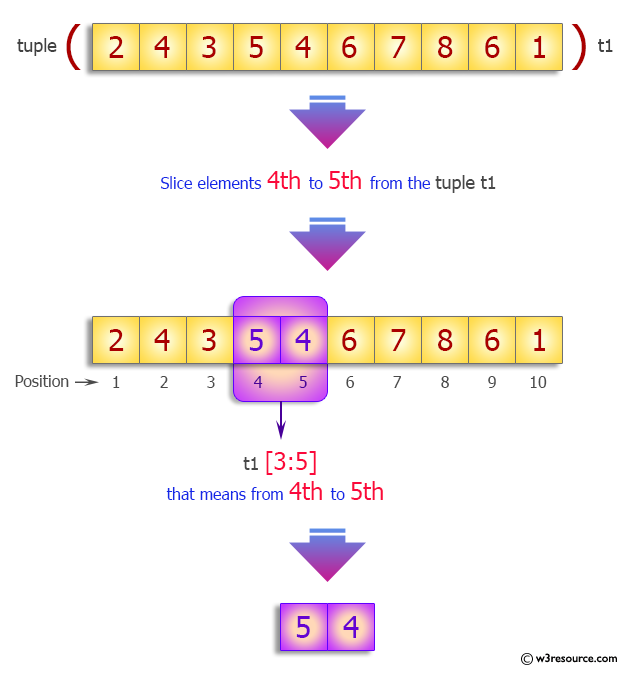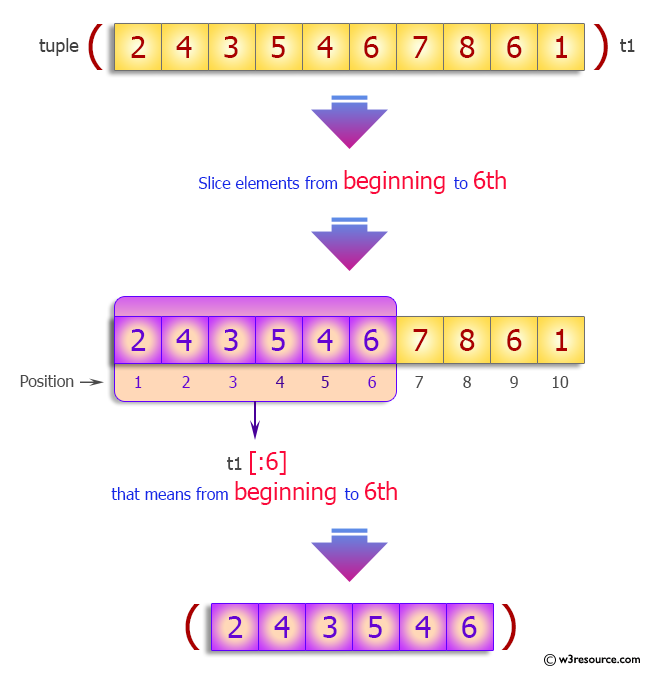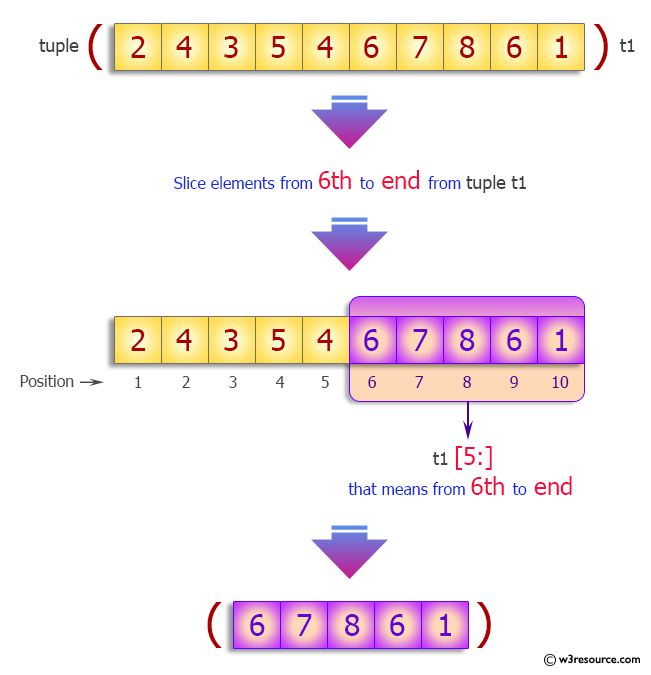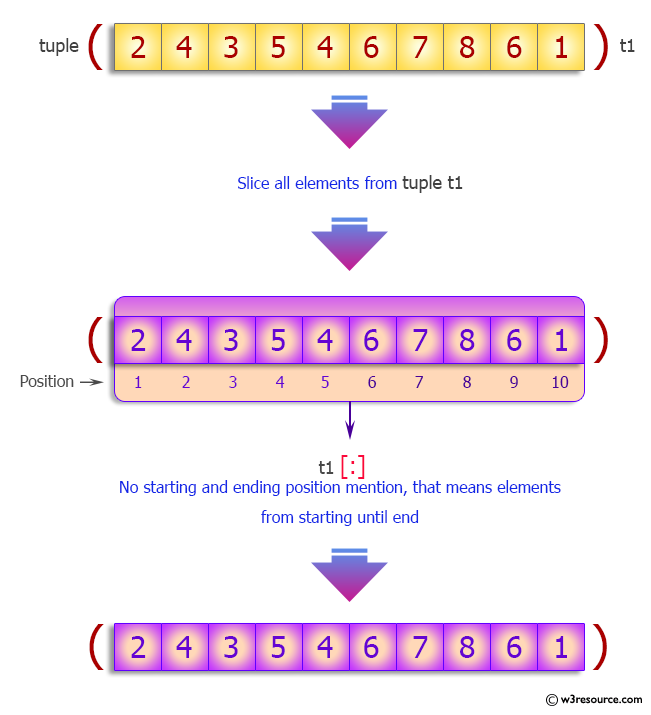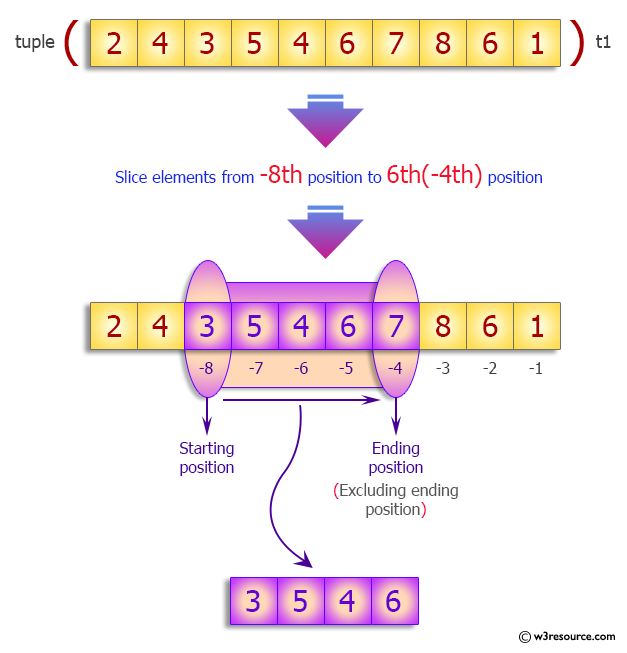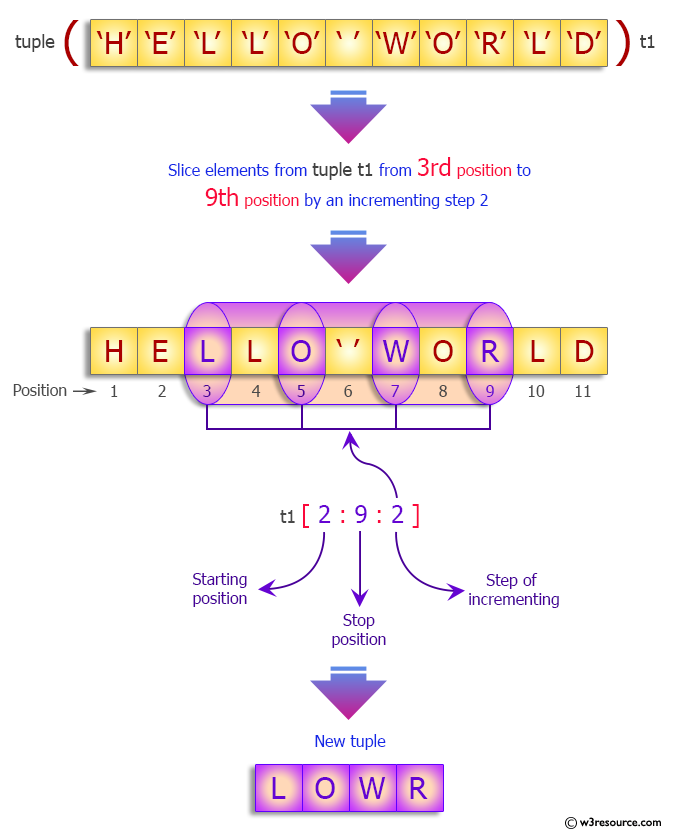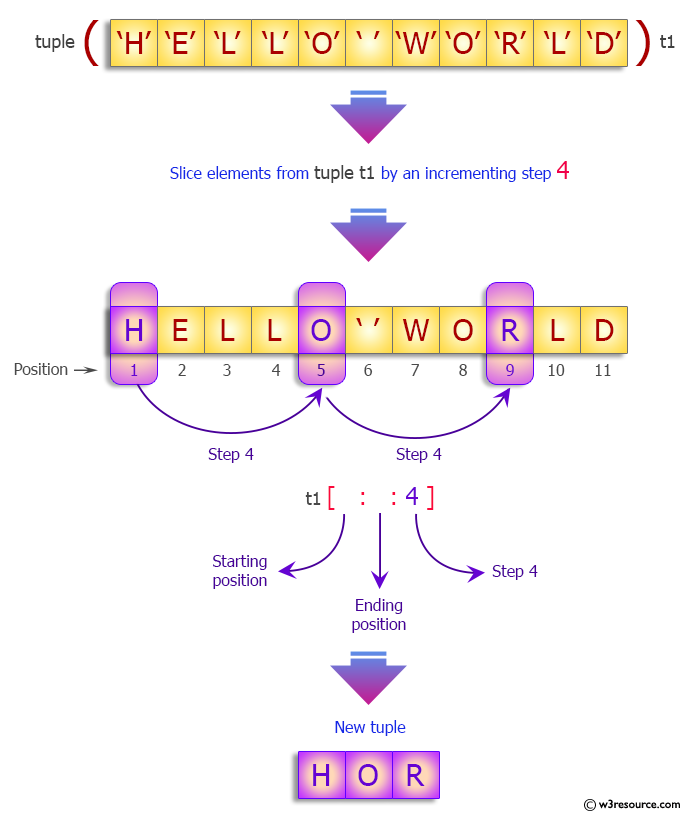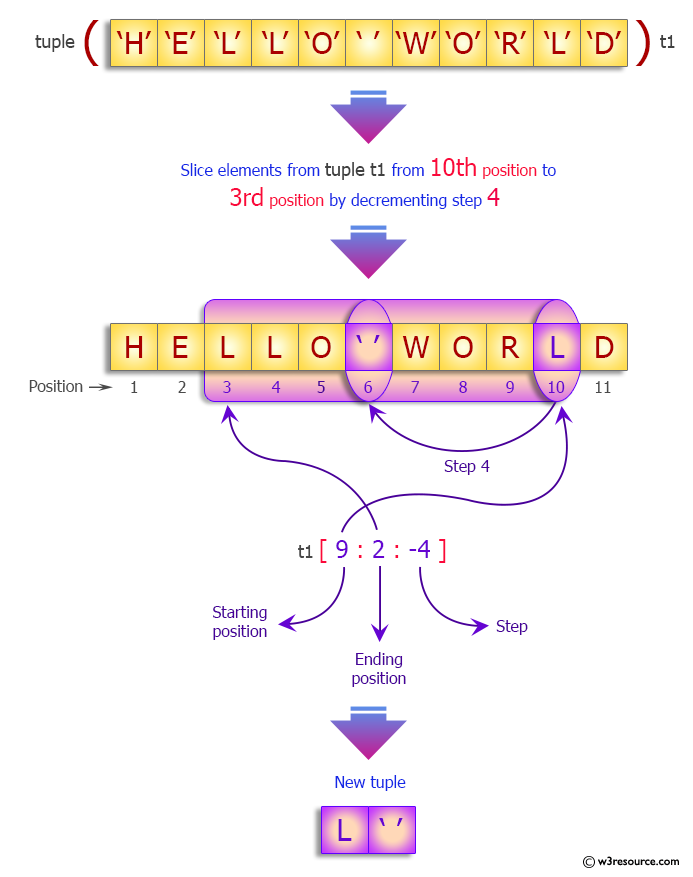Flowchart: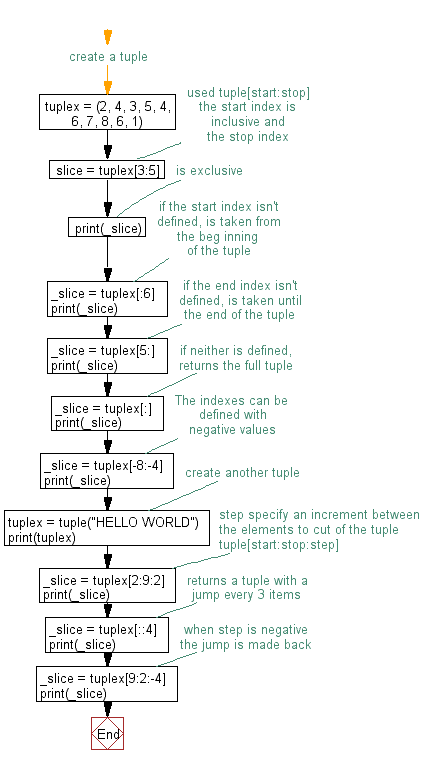Python Code Editor:

Have another way to solve this solution? Contribute your code (and comments) through Disqus.

What is the difficulty level of this exercise?

Test your Programming skills with w3resource's quiz.

﻿

## Python: Tips of the Day

How do I check if a list is empty?

For example, if passed the following:

```a = []
if not a:
print("List is empty")
```

Ref: https://bit.ly/2A4JXx9